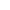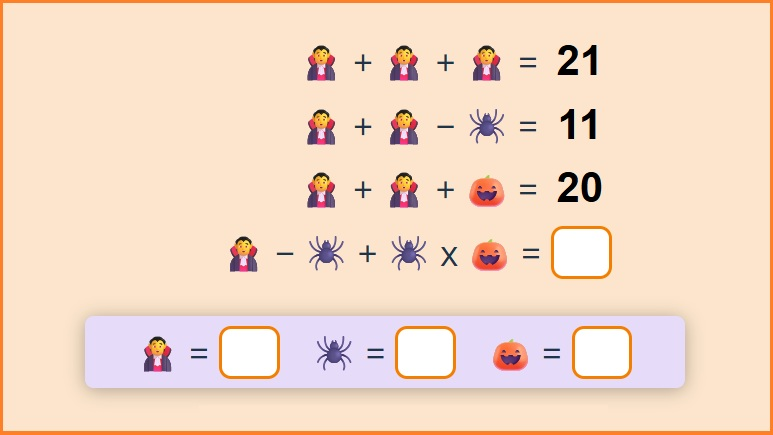﻿ Math Halloween Puzzles Online + PDF 🎃

Page No. 1034

# Math Halloween Puzzles

Math Halloween puzzles games. Fun Halloween math activities. Online halloween math games printable. Math Halloween logic puzzles. Halloween math puzzles games. Free printable Hallowen emoji puzzles. Interactive halloween math games online. Mashup math Halloween games puzzles. Math playground Halloween math logic puzzles.

New gameSolve math Halloween puzzles online.

## Math Halloween Puzzles OnlineThese math Halloween puzzles games are generated automatically. Just click on the worksheet and a new Halloween emoji puzzle in PDF will be created.

## Free Halloween math games PRINTABLE

If you are looking for more math learning ideas for including kids' excitement into your daily teaching, try some of these math Halloween puzzles for a quick and easy warm-up to start the day.

These Halloween math activities are super engaging and loved by students. You can find all Halloween math worksheets here.

## Halloween logic puzles

Enjoy the fun Halloween time and play our math Halloweeen puzzles online. These Halloween emoji puzzles challenge kids to use their logic and reasoning skills to find the value of 3 different symbols and to solve the math equation on the last line.

You can choose the difficulty level of our interactive Halloween math games:

• ### Easy Halloween logic puzles

Level 1: The easiest Halloween emoji puzzles help students to learn basic equations. Because these Halloween math puzzles don´t contain multiplication they are most useful for 2nd graders.

• ### Halloween emoji puzzles incl. basic multiplication

Level 2: Medium difficulty level. These online Halloween games are appointed for kids who already started with multiplication. When the equation does not contain parentheses, multiplication takes precedence over addition and subtraction.

• ### Hard Halloween puzzles

Level 3: Halloween math logic puzzles for 3rd graders and up. These math Halloween games contain multiplication and division problems.

Halloween math puzzles involve problem-solving, which can help students develop critical thinking skills and learn to approach problems in a logical and systematic way. Learn how to solve this type of Halloween logic puzzles in this tutorial on YouTube.

## Math Halloween puzzles games

I’m a huge fan of online math games as a way of bringing a little fun into math lessons.

And these Halloween puzzles games keep kids indeed engaged.

These Halloween math activities practice addition and subtraction along with basic multiplication and division.## Halloween math puzles

Recent studies show that working on logic math puzzles (as these Halloween emoji puzzles) leads to significant improvements in math performance.

Check also other Non-Halloween tricky maths puzzles here.

## Interactive Halloween math games and puzzles

These Halloween math games can be used by any device (Mac, PC, tablet or mobile phone) as long as you have an internet connection. You can share a direct link with your students in whatever learning system you use. The Halloween games are self-checking and give feedback to the student.

### Halloween math logic puzzles

Math Halloween puzzles can be a useful and enjoyable way to help students learn and practice math while also celebrating Halloween.

And these kinds of Halloween math logic puzzles are awesome for giving your students an opportunity to think creatively, logically and outside-of-the-box.

## Halloween math puzles PRINTABLE

I am very happy that you enjoy these interactive Halloween math games. But sometimes we need a break from computers. Therefore, I also created free printable math Halloween puzzles in PDF. While they are generated automatically you can have as many Halloween themed math worksheets as you want.

## Halloween math activities

If you are looking for some Halloween math activities to mix up your lesson plans and boost engagement, you can use these math Halloween puzzles online. These math puzzles are differentiated based on grade and ability levels, so every student can find math Halloween emoji puzzle that suits him/her best.

### Math Halloween puzzles

These Halloween math puzzles are here for you if you're looking for a simple way to squeeze in a little extra math practice to keep your kids' minds sharp over Halloween days.